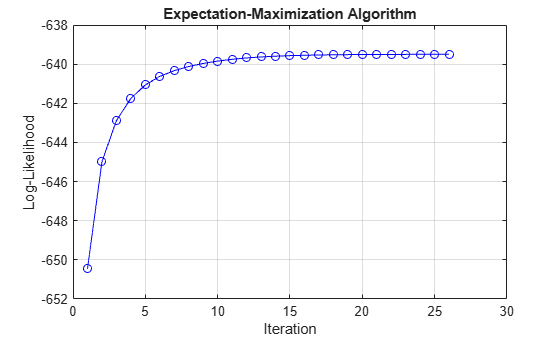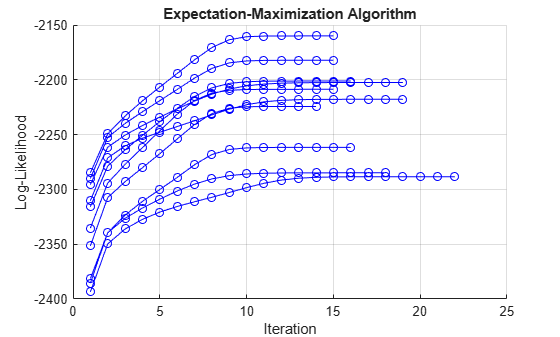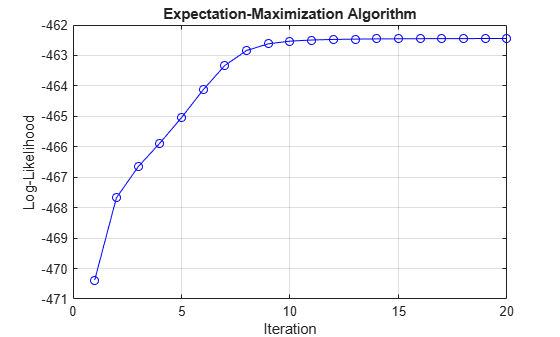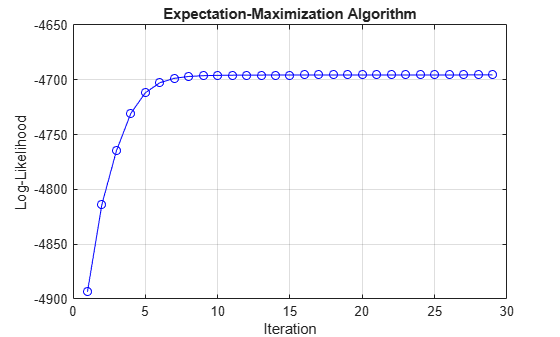# estimate

Fit Markov-switching dynamic regression model to data

## Syntax

``EstMdl = estimate(Mdl,Mdl0,Y)``
``EstMdl = estimate(Mdl,Mdl0,Y,Name,Value)``
``````[EstMdl,SS,logL] = estimate(___)``````
``````[EstMdl,SS,logL] = estimate(ax,___)``````
``````[EstMdl,SS,logL,h] = estimate(ax,___)``````

## Description

example

``EstMdl = estimate(Mdl,Mdl0,Y)` estimates parameters of the Markov-switching dynamic regression model `Mdl`. `estimate` fits the model to the response data `Y`, and initializes the estimation procedure by treating the parameter values of the fully specified Markov-switching dynamic regression model `Mdl0` as initial values. `estimate` uses a version of the expectation-maximization (EM) algorithm described by Hamilton .`

example

````EstMdl = estimate(Mdl,Mdl0,Y,Name,Value)` uses additional options specified by one or more name-value pair arguments. For example, `'IterationPlot',true` displays a plot of the loglikelihood versus the iteration step, after the algorithm terminates.```

example

``````[EstMdl,SS,logL] = estimate(___)``` also returns expected smoothed state probabilities `SS` and the estimated loglikelihood `logL` when the algorithm terminates, using any of the input argument combinations in the previous syntaxes.```
``````[EstMdl,SS,logL] = estimate(ax,___)``` plots on the axes specified by `ax` instead of the current axes (`gca`). The option `ax` can precede any of the input argument combinations in the previous syntaxes.```
``````[EstMdl,SS,logL,h] = estimate(ax,___)``` also returns the plot handle `h`. Use `h` to modify properties of the plot after you create it.```

## Examples

collapse all

Consider a two-state Markov-switching dynamic regression model of the postwar US real GDP growth rate, as estimated in .

Create Partially Specified Model for Estimation

Create a Markov-switching dynamic regression model for the naive estimator by specifying a two-state discrete-time Markov chain with an unknown transition matrix and AR(0) (constant only) submodels for both regimes. Label the regimes.

```P = NaN(2); mc = dtmc(P,'StateNames',["Expansion" "Recession"]); mdl = arima(0,0,0); Mdl = msVAR(mc,[mdl; mdl]);```

`Mdl` is a partially specified `msVAR` object. `NaN`-valued elements of the `Switch` and `SubModels` properties indicate estimable parameters.

Create Fully Specified Model Containing Initial Values

The estimation procedure requires initial values for all estimable parameters. Create a fully specified Markov-switching dynamic regression model that has the same structure as `Mdl`, but set all estimable parameters to initial values. This example uses arbitrary initial values.

```P0 = 0.5*ones(2); mc0 = dtmc(P0,'StateNames',Mdl.StateNames); mdl01 = arima('Constant',1,'Variance',1); mdl02 = arima('Constant',-1,'Variance',1); Mdl0 = msVAR(mc0,[mdl01; mdl02]);```

`Mdl0` is a fully specified `msVAR` object.

Load the US GDP data set.

`load Data_GDP`

`Data` contains quarterly measurements of the US real GDP in the period 1947:Q1–2005:Q2. The estimation period in  is 1947:Q2–2004:Q2. For more details on the data set, enter `Description` at the command line.

Transform the data to an annualized rate series by:

1. Converting the data to a quarterly rate within the estimation period

2. Annualizing the quarterly rates

```qrate = diff(Data(2:230))./Data(2:229); % Quarterly rate arate = 100*((1 + qrate).^4 - 1); % Annualized rate```

Estimate Model

Fit the model `Mdl` to the annualized rate series `arate`. Specify `Mdl0` as the model containing the initial estimable parameter values.

`EstMdl = estimate(Mdl,Mdl0,arate);`

`EstMdl` is an estimated (fully specified) Markov-switching dynamic regression model. `EstMdl.Switch` is an estimated discrete-time Markov chain model (`dtmc` object), and `EstMdl.Submodels` is a vector of estimated univariate VAR(0) models (`varm` objects).

Display the estimated state-specific dynamic models.

`EstMdlExp = EstMdl.Submodels(1)`
```EstMdlExp = varm with properties: Description: "ARIMA(0,0,0) Model (Gaussian Distribution)" SeriesNames: "Y1" NumSeries: 1 P: 0 Constant: 4.90146 AR: {} Trend: 0 Beta: [1×0 matrix] Covariance: 12.087 1-Dimensional VAR(0) Model ```
`EstMdlRec = EstMdl.Submodels(2)`
```EstMdlRec = varm with properties: Description: "ARIMA(0,0,0) Model (Gaussian Distribution)" SeriesNames: "Y1" NumSeries: 1 P: 0 Constant: 0.0084884 AR: {} Trend: 0 Beta: [1×0 matrix] Covariance: 12.6876 1-Dimensional VAR(0) Model ```

Display the estimated state transition matrix.

`EstP = EstMdl.Switch.P`
```EstP = 2×2 0.9088 0.0912 0.2303 0.7697 ```

Consider the model and data in Estimate Markov-Switching Dynamic Regression Model.

Create the partially specified model for estimation.

```P = NaN(2); mc = dtmc(P,'StateNames',["Expansion" "Recession"]); mdl = arima(0,0,0); Mdl = msVAR(mc,[mdl; mdl]);```

Create the fully specified model containing initial parameter values for the estimation procedure.

```P0 = 0.5*ones(2); mc0 = dtmc(P0,'StateNames',Mdl.StateNames); mdl01 = arima('Constant',1,'Variance',1); mdl02 = arima('Constant',-1,'Variance',1); Mdl0 = msVAR(mc0,[mdl01; mdl02]);```

```load Data_GDP qrate = diff(Data(2:230))./Data(2:229); arate = 100*((1 + qrate).^4 - 1); ```

Fit the model to the data. Plot the loglikelihood versus the iteration step when the estimation procedure terminates.

`EstMdl = estimate(Mdl,Mdl0,arate,'IterationPlot',true);`Assess estimation accuracy using simulated data from a known data-generating process (DGP). This example uses arbitrary parameter values.

Create Model for DGP

Create a fully specified, two-state discrete-time Markov chain model for the switching mechanism.

```P = [0.7 0.3; 0.1 0.9]; mc = dtmc(P);```

For each state, create a fully specified AR(1) model for the response process.

```% Constants C1 = 5; C2 = -2; % Autoregression coefficients AR1 = 0.4; AR2 = 0.2; % Variances V1 = 4; V2 = 2; % AR Submodels dgp1 = arima('Constant',C1,'AR',AR1,'Variance',V1); dgp2 = arima('Constant',C2,'AR',AR2,'Variance',V2);```

Create a fully specified Markov-switching dynamic regression model for the DGP.

`DGP = msVAR(mc,[dgp1,dgp2]);`

Simulate Response Paths from DGP

Generate 10 random response paths of length 1000 from the DGP.

```rng(1); % For reproducibility N = 10; n = 1000; Data = simulate(DGP,n,'Numpaths',N);```

`Data` is a 1000-by-10 matrix of simulated responses.

Create Model for Estimation

Create a partially specified Markov-switching dynamic regression model that has the same structure as the data-generating process, but specify an unknown transition matrix and unknown submodel coefficients.

```PEst = NaN(2); mcEst = dtmc(PEst); mdl = arima(1,0,0); Mdl = msVAR(mcEst,[mdl; mdl]);```

Create Model Containing Initial Values

Create a fully specified Markov-switching dynamic regression model that has the same structure as `Mdl`, but set all estimable parameters to initial values.

```P0 = 0.5*ones(2); mc0 = dtmc(P0); mdl01 = arima('Constant',1,'AR',0.5,'Variance',2); mdl02 = arima('Constant',-1,'AR',0.5,'Variance',1); Mdl0 = msVAR(mc0,[mdl01,mdl02]);```

Estimate Models

Fit the model to each simulated path. For each path, plot the loglikelihood at each iteration of the EM algorithm.

```c1 = zeros(N,1); c2 = zeros(N,1); v1 = zeros(N,1); v2 = zeros(N,1); ar1 = zeros(N,1); ar2 = zeros(N,1); PStack = zeros(2,2,N); figure hold on for i = 1:N EstModel = estimate(Mdl,Mdl0,Data(:,i),'IterationPlot',true); c1(i) = EstModel.Submodels(1).Constant; c2(i) = EstModel.Submodels(2).Constant; v1(i) = EstModel.Submodels(1).Covariance; v2(i) = EstModel.Submodels(2).Covariance; ar1(i) = EstModel.Submodels(1).AR{1}; ar2(i) = EstModel.Submodels(2).AR{1}; PStack(:,:,i) = EstModel.Switch.P; end hold off```Assess Accuracy

Compute the Monte Carlo mean of each estimated parameter.

```c1Mean = mean(c1); c2Mean = mean(c2); v1Mean = mean(v1); v2Mean = mean(v2); ar1Mean = mean(ar1); ar2Mean = mean(ar2); PMean = mean(PStack,3);```

Compare population parameters to the corresponding Monte Carlo estimates.

```DGPvsEstimate = [... C1 c1Mean C2 c2Mean V1 v1Mean V2 v2Mean AR1 ar1Mean AR2 ar2Mean]```
```DGPvsEstimate = 6×2 5.0000 5.0260 -2.0000 -1.9615 4.0000 3.9710 2.0000 1.9903 0.4000 0.4061 0.2000 0.2017 ```
`P`
```P = 2×2 0.7000 0.3000 0.1000 0.9000 ```
`PEstimate = PMean`
```PEstimate = 2×2 0.7065 0.2935 0.1023 0.8977 ```

Consider the data in Estimate Markov-Switching Dynamic Regression Model, but assume that the period of interest is 1960:Q1–2004:Q2. Also, consider adding an autoregressive term to each submodel.

Create a partially specified Markov-switching dynamic regression model for estimation. Specify AR(1) submodels.

```P = NaN(2); mc = dtmc(P,'StateNames',["Expansion" "Recession"]); mdl = arima(1,0,0); Mdl = msVAR(mc,[mdl; mdl]);```

Because the submodels are AR(1), each requires one presample observation to initialize its dynamic component for estimation.

Create the model containing initial parameter values for the estimation procedure.

```P0 = 0.5*ones(2); mc0 = dtmc(P0,'StateNames',Mdl.StateNames); mdl01 = arima('Constant',1,'Variance',1,'AR',0.001); mdl02 = arima('Constant',-1,'Variance',1,'AR',0.001); Mdl0 = msVAR(mc0,[mdl01; mdl02]);```

Load the data. Transform the entire set to an annualized rate series.

```load Data_GDP qrate = diff(Data)./Data(1:(end - 1)); arate = 100*((1 + qrate).^4 - 1);```

Identify the presample and estimation sample periods using the dates associated with the annualized rate series. Because the transformation applies the first difference, you must drop the first observation date from the original sample.

```dates = datetime(dates(2:end),'ConvertFrom','datenum',... 'Format','yyyy:QQQ','Locale','en_US'); estPrd = datetime(["1960:Q2" "2004:Q2"],'InputFormat','yyyy:QQQ',... 'Format','yyyy:QQQ','Locale','en_US'); idxEst = isbetween(dates,estPrd(1),estPrd(2)); idxPre = dates < estPrd(1); % The presample is the previous quarter.```

Fit the model to the estimation sample data. Specify the presample observation and plot the loglikelihood at each iteration when the estimation procedure terminates.

```EstMdl = estimate(Mdl,Mdl0,arate(idxEst),'Y0',arate(idxPre),... 'IterationPlot',true);```Consider the model and data in Estimate Markov-Switching Dynamic Regression Model.

Create the partially specified model for estimation.

```P = NaN(2); mc = dtmc(P,'StateNames',["Expansion" "Recession"]); mdl = arima(0,0,0); Mdl = msVAR(mc,[mdl; mdl]);```

Create the fully specified model containing initial parameter values for the estimation procedure.

```P0 = 0.5*ones(2); mc0 = dtmc(P0,'StateNames',Mdl.StateNames); mdl01 = arima('Constant',1,'Variance',1); mdl02 = arima('Constant',-1,'Variance',1); Mdl0 = msVAR(mc0,[mdl01; mdl02]);```

```load Data_GDP qrate = diff(Data(2:230))./Data(2:229); arate = 100*((1 + qrate).^4 - 1); ```

Fit the model to the data. Return the expected smoothed state probabilities and loglikelihood when the algorithm terminates.

`[EstMdl,SS,logL] = estimate(Mdl,Mdl0,arate);`

`SS` is a 228-by-2 matrix of expected smoothed state probabilities; rows correspond to periods in the estimation sample, and columns correspond to the regimes. `logL` is the final loglikelihood.

Display the expected smoothed state probabilities for the last period in the estimation sample, and display the final loglikelihood.

`SS(end,:)`
```ans = 1×2 0.8985 0.1015 ```
`logL`
```logL = -639.4962 ```

Fit simulated data to a Markov-switching dynamic regression model with VARX submodels. Specify equality constraints for estimation. This example uses arbitrary parameter values.

Create Model for DGP

Create a fully specified, three-state discrete-time Markov chain model for the switching mechanism.

```P = [0.8 0.1 0.1; 0.2 0.6 0.2; 0 0.1 0.9]; mc = dtmc(P);```

For each state, create a fully specified VARX(1) model for the response process. Specify the same model constant and lag 1 AR coefficient matrix for all submodels. For each model, specify a different 2-by-1 regression coefficient for the one exogenous variable.

```% Constants C = [1;-1]; % Autoregression coefficients AR = {[0.6 0.1; 0.4 0.2]}; % Regression coefficients Beta1 = [0.2;-0.4]; Beta2 = [0.6;-1.0]; Beta3 = [0.9;-1.3]; % VAR Submodels dgp = varm('Constant',C,'AR',AR,'Covariance',5*eye(2)); dgp1 = dgp; dgp1.Beta = Beta1; dgp2 = dgp; dgp2.Beta = Beta2; dgp3 = dgp; dgp3.Beta = Beta3; ```

Create a fully specified Markov-switching dynamic regression model for the DGP.

`DGP = msVAR(mc,[dgp1; dgp2; dgp3]);`

Simulate Data from DGP

Simulate data for the exogenous series by generating 1000 observations from the Gaussian distribution with mean 0 and variance 100.

```rng(1); % For reproducibility X = 10*randn(1000,1);```

Generate a random response path of length 1000 from the DGP. Specify the simulated exogenous data for the submodel regression components.

`Data = simulate(DGP,1000,'X',X);`

`Data` is a 1000-by-1 vector of simulated responses.

Create Model for Estimation

Create a partially specified Markov-switching dynamic regression model that has the same structure as the data-generating process, but specify an unknown transition matrix and unknown regression coefficients. Specify the true values of the constants and AR coefficient matrices.

```PEst = NaN(3); mcEst = dtmc(PEst); mdl = varm(2,1); mdl.Constant = C; mdl.AR = AR; mdl.Beta = NaN(2,1); Mdl = msVAR(mcEst,[mdl; mdl; mdl]);```

Because the values of the constants and AR coefficient matrices are specified in `Mdl`, `estimate` treats them as equality constraints for estimation.

Create Model Containing Initial Values

Create a fully specified Markov-switching dynamic regression model that has the same structure as `Mdl`, but set all estimable parameters to initial values and set parameters with equality constraints to their values specified in `Mdl`.

```P0 = (1/3)*ones(3); mc0 = dtmc(P0); mdl0 = varm('Constant',C,'AR',AR,'Covariance',eye(2)); mdl01 = mdl0; mdl01.Beta = [0.1;-0.1]; mdl02 = mdl0; mdl02.Beta = [0.5;-0.5]; mdl03 = mdl0; mdl03.Beta = [1;-1]; Mdl0 = msVAR(mc0,[mdl01; mdl02; mdl03]);```

Estimate Model

Fit the model to the simulated data. Specify the exogenous data for the regression component. Plot the loglikelihood at each iteration of the EM algorithm.

```figure EstMdl = estimate(Mdl,Mdl0,Data,'X',X,'IterationPlot',true);```Assess Accuracy

Compare the estimated regression coefficient vectors and transition matrix to their true values.

`Beta1`
```Beta1 = 2×1 0.2000 -0.4000 ```
`Beta1Estimate = EstMdl.Submodels(1).Beta`
```Beta1Estimate = 2×1 0.1596 -0.4040 ```
`Beta2`
```Beta2 = 2×1 0.6000 -1.0000 ```
`Beta2Estimate = EstMdl.Submodels(2).Beta`
```Beta2Estimate = 2×1 0.5888 -0.9771 ```
`Beta3`
```Beta3 = 2×1 0.9000 -1.3000 ```
`Beta3Estimate = EstMdl.Submodels(3).Beta`
```Beta3Estimate = 2×1 0.8987 -1.2991 ```
`P`
```P = 3×3 0.8000 0.1000 0.1000 0.2000 0.6000 0.2000 0 0.1000 0.9000 ```
`PEstimate = EstMdl.Switch.P`
```PEstimate = 3×3 0.7787 0.0856 0.1357 0.1366 0.6906 0.1727 0.0086 0.0787 0.9127 ```

## Input Arguments

collapse all

Partially specified Markov-switching dynamic regression model used to indicate constrained and estimable model parameters, specified as an `msVAR` model object returned by `msVAR`. Properties of `Mdl` describe the model structure and specify the parameters.

`estimate` treats specified parameters as equality constraints during estimation.

`estimate` fits unspecified (`NaN`-valued) parameters to the data `Y`.

Fully specified Markov-switching dynamic regression model used to initialize the estimation procedure, specified as an `msVAR` model object returned by `msVAR`. Properties of a fully specified model object do not contain `NaN` values.

`Mdl0` is a copy of `Mdl` with `NaN` values replaced by initial values.

Tip

For broad coverage of the parameter space, run the algorithm from multiple instances of `Mdl0`. For more details, see Algorithms.

Observed response data to which `estimate` fits the model, specified as a `numObs`-by-`numSeries` numeric matrix.

`numObs` is the sample size. `numSeries` is the number of response variables (`Mdl.NumSeries`).

Rows correspond to observations, and the last row contains the latest observation. Columns correspond to individual response variables.

`Y` represents the continuation of the presample response series in `Y0`.

Data Types: `double`

Axes on which to plot the loglikelihood for each iteration when `IterationPlot` is `true`, specified as an `Axes` object.

By default, `estimate` plots to the current axes (`gca`).

If `IterationPlot` is false, `estimate` ignores `ax`.

### Name-Value Pair Arguments

Specify optional comma-separated pairs of `Name,Value` arguments. `Name` is the argument name and `Value` is the corresponding value. `Name` must appear inside quotes. You can specify several name and value pair arguments in any order as `Name1,Value1,...,NameN,ValueN`.

Example: `'Y0',Y0,'X',X,'IterationPlot',true` uses the matrix `Y0` as presample responses required for estimation, includes a linear regression component in each submodel composed of the predictor data in `X` and the specified regression coefficients, and plots the loglikelihood for each iteration.

Presample response data, specified as the comma-separated pair consisting of `'Y0'` and a `numPreSampleObs`-by-`numSeries` numeric matrix.

The number of presample observations `numPreSampleObs` must be sufficient to initialize the AR terms of all submodels. If `numPreSampleObs` exceeds the AR order of any state, `estimate` uses the latest observations. By default, `Y0` is the initial segment of `Y`, which reduces the effective sample size.

Data Types: `double`

Initial state probabilities, specified as the comma-separated pair consisting of `'S0'` and a nonnegative numeric vector of length `numStates`.

`estimate` normalizes `S0` to produce a distribution.

By default, `S0` is a steady-state distribution computed by `asymptotics`.

`estimate` updates `S0` at each iteration using expected smoothed probabilities for the initial distribution.

Example: `'S0',[0.2 0.2 0.6]`

Example: `'S0',[0 1]` specifies state 2 as the initial state.

Data Types: `double`

Predictor data used to evaluate regression components in all submodels of `Mdl`, specified as the comma-separated pair consisting of `'X'` and a numeric matrix or a cell vector of numeric matrices.

To use a subset of the same predictors in each state, specify `X` as a matrix with `numPreds` columns and at least `numObs` rows. Columns correspond to distinct predictor variables. Submodels use initial columns of the associated matrix, in order, up to the number of submodel predictors. The number of columns in the `Beta` property of `Mdl.SubModels(j)` determines the number of exogenous variables in the regression component of submodel `j`. If the number of rows exceeds `numObs`, then `estimate` uses the latest observations.

To use different predictors in each state, specify a cell vector of such matrices with length `numStates`.

By default, `estimate` ignores regression components in `Mdl`.

Data Types: `double`

Convergence tolerance, specified as the comma-separated pair consisting of `'Tolerance'` and a positive numeric scalar.

The EM algorithm terminates after an iteration in which the loglikelihood `logL` changes by less than `Tolerance`.

Example: `'Tolerance',1e-6`

Data Types: `double`

Maximum number of iterations of the EM algorithm, specified as the comma-separated pair consisting of `'MaxIterations'` and a positive integer.

Example: `'MaxIterations',1000`

Data Types: `double`

Flag for plotting the loglikelihood at each iteration, specified as the comma-separated pair consisting of `'IterationPlot'` and a value in this table.

ValueDescription
`true`When the algorithm terminates, `estimate` shows a plot of the loglikelihood at each iteration step.
`false``estimate` does not show a plot.

Example: `'IterationPlot',true`

Data Types: `logical`

## Output Arguments

collapse all

Estimated Markov-switching dynamic regression model, returned as an `msVAR` object. `EstMdl` is a copy of `Mdl` that has `NaN` values replaced with parameter estimates. `EstMdl` is fully specified.

Note

Row normalization can alter specified values in the transition matrix of the input model `Mdl.Switch.P`.

Expected smoothed state probabilities when the algorithm terminates, returned as a `numObs`-by-`numStates` numeric matrix.

Estimated loglikelihood of the response data `Y` with respect to the estimated model `EstMdl` when the algorithm terminates, returned as a numeric scalar.

Handle to the iteration plot, returned as a graphics object when `IterationPlot` is `true`.

`h` contains a unique plot identifier, which you can use to query or modify properties of the plot.

## Tips

• In , Hamilton cautions: "Although a researcher might be tempted to use the most general specification possible, with all the parameters changing across a large number of regimes...in practice this is usually asking more than the data can deliver." Hamilton recommends model parsimony and selective estimation, to "limit the focus to a few of the most important parameters that are likely to change."

• Data-generating processes with low variances can lead to difficulties in state inference and subsequent parameter estimation. In such cases, consider scaling the data. The variance scales quadratically.

## Algorithms

• `estimate` implements a version of the EM algorithm described in , , and . Given an initial estimate of model parameters `Mdl0`, `estimate` iterates the following process until convergence:

1. Expectation step — `estimate` applies `smooth` to the data to obtain inferences of latent state probabilities at each time step and an estimate of the overall data loglikelihood.

2. Maximization step — `estimate` uses the expected smoothed probabilities from the expectation step to weight the data before updating parameter estimates in each submodel.

• Likelihood surfaces for the mixture densities of switching models can contain local maxima and singularities . If so, the largest local maximum with a nonzero model variance is considered the maximum likelihood estimate (MLE). If `Mdl0` is in the neighborhood of the MLE, `estimate` typically converges to it, but this convergence is not guaranteed.

• `estimate` handles two types of constraints:

• Constraints on submodel parameters, which the `estimate` object function of the `varm` object enforces at each maximization step

• Constraints on transition probabilities, which `estimate` enforces by projecting a maximal estimate of the transition matrix onto the constrained parameter space after each iteration

Constraints on submodel innovations variances and covariances are unsupported. `estimate` computes innovations covariances after estimation, regardless of their values.

 Chauvet, M., and J. D. Hamilton. "Dating Business Cycle Turning Points." In Nonlinear Analysis of Business Cycles (Contributions to Economic Analysis, Volume 276). (C. Milas, P. Rothman, and D. van Dijk, eds.). Amsterdam: Emerald Group Publishing Limited, 2006.

 Hamilton, J. D. "Analysis of Time Series Subject to Changes in Regime." Journal of Econometrics. Vol. 45, 1990, pp. 39–70.

 Hamilton, James D. Time Series Analysis. Princeton, NJ: Princeton University Press, 1994.

 Hamilton, J. D. "Macroeconomic Regimes and Regime Shifts." In Handbook of Macroeconomics. (H. Uhlig and J. Taylor, eds.). Amsterdam: Elsevier, 2016.

 Kole, E. "Regime Switching Models: An Example for a Stock Market Index." Rotterdam, NL: Econometric Institute, Erasmus School of Economics, 2010.